# The Open Mathematics, Statistics and Probability Journal

The Open Statistics & Probability Journal

(Discontinued)

ISSN: 2666-1489 ― Volume 10, 2020
RESEARCH ARTICLE

# Consistency of the Semi-parametric MLE under the Cox Model with Right-Censored Data

Qiqing Yu1, *
1 Department of Mathematical Sciences, SUNY, Binghamton, NY, 13902, USA

## Abstract

### Objective:

We studied the consistency of the semi-parametric maximum likelihood estimator (SMLE) under the Cox regression model with right-censored (RC) data.

### Methods:

Consistency proofs of the MLE are often based on the Shannon-Kolmogorov inequality, which requires finite E(lnL), where L is the likelihood function.

### Results:

The results of this study show that one property of the semi-parametric MLE (SMLE) is established.

### Conclusion:

Under the Cox model with RC data, E(lnL) may not exist. We used the Kullback-Leibler information inequality in our proof.

Keywords: Cox model, Maximum likelihood estimator, Consistency, Kullback-Leibler Inequality, Shannon-Kolmogorov inequality, Without loss of generality (WLOG).

### Article Information

#### Identifiers and Pagination:

Year: 2020
Volume: 10
First Page: 21
Last Page: 27
Publisher Id: TOSPJ-10-21
DOI: 10.2174/2666148902010010021

#### Article History:

Acceptance Date: 19/08/2020
Electronic publication date: 23/10/2020
Collection year: 2020

open-access license: This is an open access article distributed under the terms of the Creative Commons Attribution 4.0 International Public License (CC-BY 4.0), a copy of which is available at: https://creativecommons.org/licenses/by/4.0/legalcode. This license permits unrestricted use, distribution, and reproduction in any medium, provided the original author and source are credited.

* Address correspondence to this author at the Department of Mathematical Sciences, SUNY, Binghamton, NY, 13902, USA; E-mail: qyu@math.binghamton.edu

## 1. INTRODUCTION

We studied the consistency of the semi-parametric maximum likelihood estimator (SMLE) under the Cox model with right-censored (RC) data.

Let Y be a random survival time, X a p-dimensional random covariate. Conditional on X = x, Y satisfies the Cox model if its hazard function satisfies(1.1)

where ho is the baseline hazard function, i.e., ho (y) = fo (y) /So (y-), fo is a density function, So (y) = S(y|0)P (Y > y |X = 0), Fo = 1 - So, τY = sup{t:SY(t) > 0}, h(y|x) =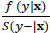, S(·|·) f(·|·) orF(·|·)) is the conditional survival function (density function (df) or cumulative distribution function (cdf)) of Y given X = x. The restriction y<τY is not in the original definition of the PH model, but is necessary if So is discontinuous at τY (see Remark 1 [1Yu. Qiqing, "A note on the proportional hazards model with discontinuous data", Stat. Probab. Lett., vol. 77, no. 7, pp. 735-739.
[http://dx.doi.org/10.1016/j.spl.2006.11.008]
])

## 2. METHODS

In this paper, we shall make use of the assumptions as follows:

AS1. Suppose that C is a random variable with the df fC (t) and the survival function SC (t), X takes at least p +1 values, say 0 , x1, ..., xp, where x1, ..., xp are linearly independent, (Y,X) and C are independent. Let (Y1,X1,C1), ..., (Yn,Xn,Cn) be i.i.d. random vectors from (Y,X,C). M = min(Y,C) and δ = 1(YC), where 1(A) is the indicator function of the event A. Let (M1, δ1X1), ..., (Mn, δn, Xn) be i.i.d. RC observations from (M, δ, X) with the df are as follows:(1.2)and S(t|x) is a function of (So, β) (see Eq. (1.1)), but not fx and fC (the df’s of X and C).

Due to (AS1) and Eq. (1.2), the generalized likelihood function can be written as: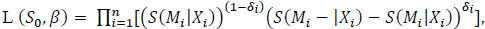(1.3)

which coincides with the standard form of the generalized likelihood [2J. Kiefer, "J and J. Wolfowitz. “Consistency of the maximum likelihood estimator in the presence of infinitely many incidental parameters", Ann. Math. Stat., vol. 1, pp. 887-906.
[http://dx.doi.org/10.1214/aoms/1177728066]
]. Eq. (1.3) is identical to the next expression:(1.4)

where ηn = min{|Mi-Mj|: MiMj, i, j{1,2, ..., n}}. This form allows So to be arbitrary (discrete or continuous, or others), thus is more convenient in the later proofs. If Y is continuous then S(t|x) = (S(t|0))exp(X'β) = (So (t))exp(X'β), but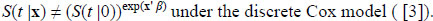(1.5)

If Y is discrete then S(t|x) = ∏s≤t(1 - h(s|x)) = ∏s≤t(1 - h0 (s)eX'β) If Y has a mixture distribution, then S(t|x)= p (S01(t))exp(X'β) + (1 - p) ∏s≤t(1 - h02(s)eX'β where p(0,1), h01 and h02 are two hazard functions. h0 (t) = ph01 + (1 - p)h02 and S0 (t) = pS01 + (1 - p)S02

The SMLE of (So, β) maximizes L (S, b) overall possible survival function S and bRp, denoted by (). The SMLE of S(t|x) is denoted by(t|x), which is a function of (). The computation issue of the SMLE under the Cox model has been studied, but its consistency has not been established under the model [3G.Y.C. Wong, M.P. Osborne, Q.G. Diao, and Q.Q. Yu, "The piece-wise cox model with right-censored data", Comm. Statist. Comput. Simul., vol. 46, pp. 7894-7908.
[http://dx.doi.org/10.1080/03610918.2016.1255968]
]. Their simulation results suggest that the SMLE is more efficient than the partial likelihood estimator under the Cox model.

The partial likelihood estimator is a common estimator under the Cox model, which maximizes the partial likelihood:, where D is the collection of indices of the exact observations and Ri is the risk set {j: MjYj}. The asymptotic properties of the estimator are well understood [4D.R. Cox, and D. Oakes, Analysis of Survival Data., Chapman & Hall NY, .].

The consistency of the SMLE under the continuous Cox model with interval-censored (IC) data has been established, making use of the following result [5Q.Q. Yu, and Q.G Diao, "Consistency of the semi-parametric MLE under the Cox model with linearly time-dependent covariates and interval-censored data", J. Adv.Stat., vol. 4, no. 1, .]:

The Shannon-Kolmogorov (S-K) inequality. Let fo and f be two densities with respect to (w.r.t.) a measure μ and ∫ f0 (t)ln f0 (t)(t) is finite. Then, ∫ f0 (t)ln f0 (t)(t) ≥ ∫ f0 (t)ln f (t)(t), with equality iff f = fo a.e. w.r.t. μ.

Under the Cox model with IC data, the S-K inequality becomes E (lnL(So, β)) E (lnL(S, b))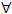(S, b), where L(∙, ∙) is the likelihood function of the Cox model with IC data, which is different from L ( ∙, ∙) in Eq. (1.3) and S is a baseline survival function and bRp. Their approach cannot be extended to the Cox model with RC data as the key assumption (in the S-K inequality) [3G.Y.C. Wong, M.P. Osborne, Q.G. Diao, and Q.Q. Yu, "The piece-wise cox model with right-censored data", Comm. Statist. Comput. Simul., vol. 46, pp. 7894-7908.
[http://dx.doi.org/10.1080/03610918.2016.1255968]
].

That is, finite E (lnL (So, β)), may not hold. Indeed, if Y has a df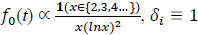and β = 0, then L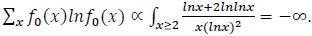A related inequality is as follows.

The Kullback-Leibler (K-L) information inequality. Let fo and f be two densities w.r.t. a measure μ. Thenf0 (t)ln (f0 /f)(t)(t) ≥ 0, with equality iff f = foa.e. w.r.t. μ.

The K-L inequality says that ∫ f0 (t)ln (f0 /f)(t)(t) exists, though it maybe . The two inequalities are not equivalent. In fact,In this note, we show that the SMLE under the Cox model is consistent, making use of the Kullback-Leibler information inequality [6S. Kullback, and R.A. Leibler, "On information and sufficiency", Ann. Math. Stat., vol. 22, pp. 79-86.
[http://dx.doi.org/10.1214/aoms/1177729694]
]

2. The Main Results. Notice that under the assumption that ho exists, So, fo, Fo and ho are equivalent, in the sense that given one of them, the other 3 functions can be derived. Thus, the Cox model is applicable only to the distributions that the density functions exist, that is, Y is either continuous, or discrete, or the mixture of the previous two. Since the expression of S(t|x) varies in these three cases, for simplicity, we only prove the consistency of the SMLE under the Cox model in the first two cases.

Theorem 1. Under the Cox model with RC data, if Y is either continuous or discrete, and ifSoM) <1, then the SMLE (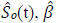) is consistenttD (see Eq. (1.2)).

The proof of Theorem 1 makes use of a modified K-L inequality. K-L inequality requires that f0 and f are both densities w.r.t. the measure μ. That is ∫ f(t)(t = 1. However, in our case, we encounter the case that ∫ f(t)(t)[0,1].

Lemma 1 (the modified K-L inequality). If fi ≥ 0, μ1 is a measure, ∫ f1(t)1(t = 1 and ∫ f2(t)1(t ≤ 1, then ∫ f1(t)ln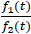1(t) ≥ 0, with equality iff f1 = f2 a.e. w.r.t. μ1.

Proof. In view of the K-L inequality, it suffices to prove the inequality ∫ f1(t)ln1(t) ≥ 0 under the additional assumptions that ∫ f2(t)1(t < 1, ∫ f1(t)2(t = 0 and ∫ f2(t)(t < 1, where μ2 is a measure and μ = μ1 + μ2 Since ∫ f2(t)(t) = 1, f1 and f2 are df's w.r.t. μ.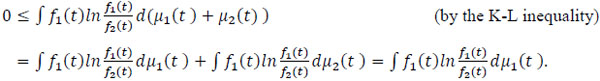Proof of Theorem 1. Let 0 be the subset of the sample space such that the empirical distribution function (edf)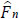, (t, s, x) based on (Mi, δi, Xi) converges to F(t,s,x), the cdf of (M, δ, X). It is well-known that P(Ω0,) =1. Notice that the SMLE () is a function of (ω, n), say (o,n (t)(ω),o,n (tn)(ω) , where ωΩ and n is the sample size. Hereafter, fix an ω0 , since(=n(ω)) is a sequence of vectors in Rp, there is a convergent subsequence with the limit β*, where the components of β* can be ±∞. Moreover, So (= So,n ()(ω)) is a sequence of bounded non-increasing functions, Helly’s selection theorem ensures that given any subsequence ofo, there exists a further subsequence which is convergent. Without loss of generality (WLOG), we assume thatoS* andβ*. Of course, (β*, S*) depends on ω(0 ). We prove in Theorem 2 for the discrete case and in Theorem 3 for the continuous case that: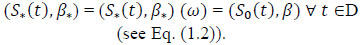(2.1)

Since ω can be arbitrary in Ω0 and P(Ω0 ) = 1, the SMLE is consistent.

Before we prove Theorems 2 and 3, we present a preliminary result.

Lemma 2 (Proposition 17 in Royden (1968), page 231). Suppose thatμn is a sequence of measures on the measurable space (J,) such that μn(B) μ(B),B, gn and fn are non-negative measurable functions, and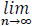(fn, gn)(x) = (f, g)(x) Then,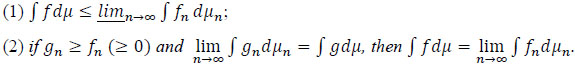Corollary 1. Suppose that μn is a sequence of measures on the measurable space (J , B) such thatμn (B) → μ (B),B, f andfn (n ≥ 1) are integrable functions that are bounded below andf(x)n→∞ = lim fn(x). Thenf dμlimn→∞fnn.

Proof. Let k = infn infxfn(x). If k ≥ 0 then the corollary follows from Lemma 2. Otherwise, let fn-(x) = 0 Λ fn(x), fn+(x) = 0 v fn(x), f-(x) = 0 Λ f(x) and f +(x) = 0 v f(x). Then, fn+f + and fn-f - point wisely, as, fnf

limn→∞fnn = limn→∞ ∫ (fn+ + fn-)n = limn→∞ [∫ fn+n + fn-n] ≥ ∫ limn→∞fn+ + ∫ limn→∞fn- (by Lemma2, as fn+ (x) is nonnegative and |f- (x)| ≤ k) = ∫ f + + ∫ f- = ∫ (f + + f-) = ∫ f dμ.

Theorem 2. Under the discrete Cox model with RC data, Eq. (2.1) holds.

Proof. For the given ω0 and (S*, β*) in the proof of Theorem 1, as assumed, () (ω) → (S*, β*). Defining h*(t) =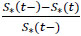and h*(t|x) = h*(t)eβ*'x (for S*(t -) > 0) yeilds S*(t|x) and f*(t|x), which are continuous functions of S* and β*. Consequently,(·|·) → S*(·|·).

Let Gn(S0 , β) = lnL(S0 , β)/n (see Eq.(1.3)). Then, the SMLE () satisfies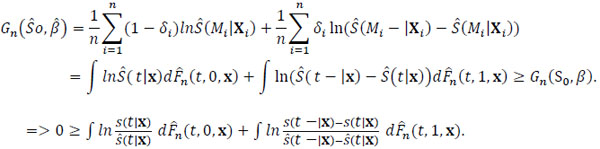(2.2).

where B is a measurable set in Rp+1. To apply Lemma 2,(2.3)(2.4)(2.5)(2.6)(2.7)(2.8)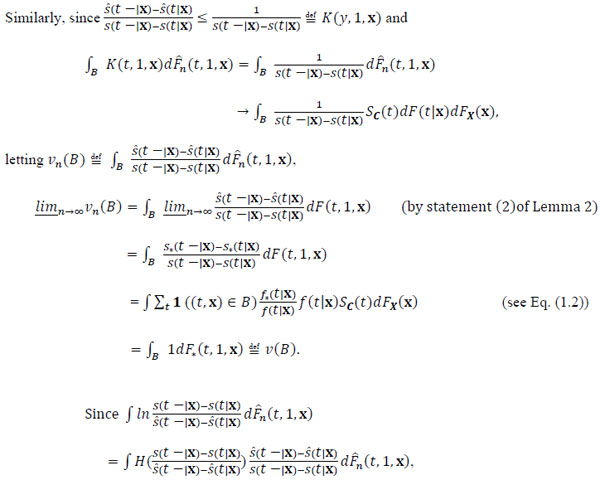(2.9)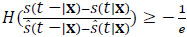and vn converges set wisely to a finite measure v (see (2.9)), by a similar argument as in (2.4), (2.6), (2.7) and (2.8), we have:(2.10)

Thus, ∫ lndF(t, 0, x) + ∫ ln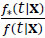dF(t, 1, x). Hence, (S0 (t),β) = (S*(t),β)tD by the 2nd statement of the K-L inequality.

Theorem 3.Under the Cox model with RC data, if Y is continuous then Eq. (2.1) holds.

Proof. For the given ω0 and (S**) in the proof of Theorem 1, as well as(ω) and(t|x)(ω), we have S*(t|x) = (S*(t))exp(β*'x). By a similar argument as in proving Eq. (2.8), we can show: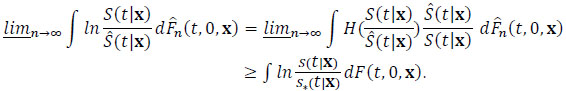(2.11)

In view of Eq. (1.4) due to Y is continuous, we denote: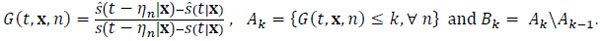(2.12)(2.13)

as S* is a monotone function, S*' exists a.e., and so do S*'(t|x) and F*'(t|x). We have(2.14)

The reason is as follows. For each (t, x) such that F'(t|x) > 0 and Eq. (2.13) holds,

F*'(t|x) /F'(t|x) (=f*(t|x) /f (t|x)) is finite. Then, there exists no such that G(t, x, n) < 1 + F*'(t|x) /F'(t|x) for nno . On the other hand, G(t, x, n) is finite for n =1, ..., no . Thus, G(t, x, n) < k for some k. Since Eq. (2.1) holds a.e. and ∫ 1dF(t, s, x) = 1, Eq. (2.14) holds.

We shall prove in Lemma 3 that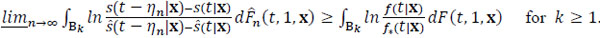(2.15)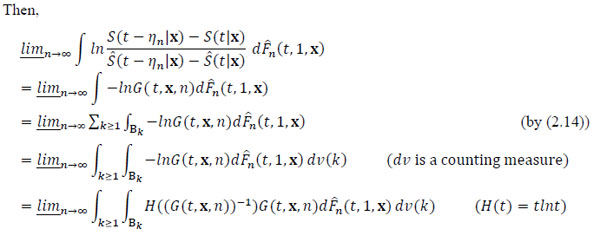.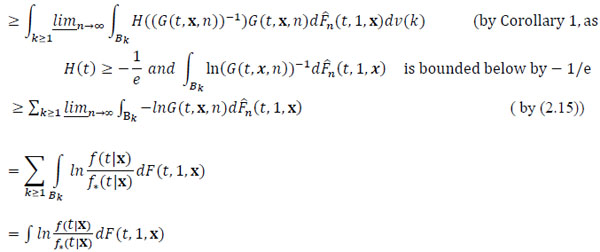(2.16).

## 3. RESULTS

The last inequality further implies that ∫lnd F(t,0,x) + ∫lnd F(t,1,x) = 0. Thus, (S0 (t),β) = (S*(t),β*)tD by the 2nd statement of the K-L inequality and by the assumption ASI.

Lemma 3. Inequality (2.15) holds.

Proof. Let k ≥ 1 and, where B is a measurable set and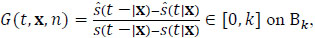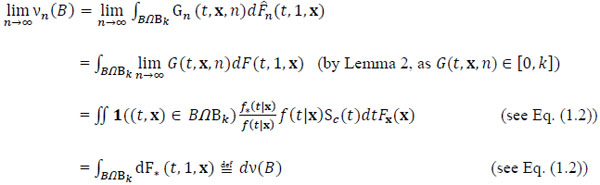.

## CONCLUSION

Since H((S (t-ηn|x) - (S (t|x))/(((t-ηn|x) - ((t|x))) ≥ - 1/e and vn converges set wisely to a finite measure v by a similar argument as in (2.4), (2.6), (2.7) and (2.8), we can show that: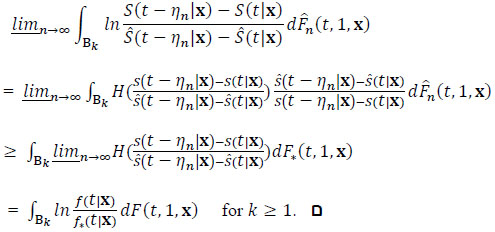.

Not applicable.

Not applicable.

None.

### CONFLICT OF INTEREST

The author declare no conflict of interest, financial or otherwise.

### ACKNOWLEDGEMENTS

The author would like to thank the editor and two referees for their invaluable comments.

## REFERENCES

  Yu. Qiqing, "A note on the proportional hazards model with discontinuous data", Stat. Probab. Lett., vol. 77, no. 7, pp. 735-739.[http://dx.doi.org/10.1016/j.spl.2006.11.008]  J. Kiefer, "J and J. Wolfowitz. “Consistency of the maximum likelihood estimator in the presence of infinitely many incidental parameters", Ann. Math. Stat., vol. 1, pp. 887-906.[http://dx.doi.org/10.1214/aoms/1177728066]  G.Y.C. Wong, M.P. Osborne, Q.G. Diao, and Q.Q. Yu, "The piece-wise cox model with right-censored data", Comm. Statist. Comput. Simul., vol. 46, pp. 7894-7908.[http://dx.doi.org/10.1080/03610918.2016.1255968]  D.R. Cox, and D. Oakes, Analysis of Survival Data., Chapman & Hall NY, .  Q.Q. Yu, and Q.G Diao, "Consistency of the semi-parametric MLE under the Cox model with linearly time-dependent covariates and interval-censored data", J. Adv.Stat., vol. 4, no. 1, .  S. Kullback, and R.A. Leibler, "On information and sufficiency", Ann. Math. Stat., vol. 22, pp. 79-86.[http://dx.doi.org/10.1214/aoms/1177729694]

### Endorsements

"Open access will revolutionize 21st century knowledge work and accelerate the diffusion of ideas and evidence that support just in time learning and the evolution of thinking in a number of disciplines."

Daniel Pesut
(Indiana University School of Nursing, USA)

"It is important that students and researchers from all over the world can have easy access to relevant, high-standard and timely scientific information. This is exactly what Open Access Journals provide and this is the reason why I support this endeavor."

Jacques Descotes
(Centre Antipoison-Centre de Pharmacovigilance, France)

"Publishing research articles is the key for future scientific progress. Open Access publishing is therefore of utmost importance for wider dissemination of information, and will help serving the best interest of the scientific community."

Patrice Talaga
(UCB S.A., Belgium)

"Open access journals are a novel concept in the medical literature. They offer accessible information to a wide variety of individuals, including physicians, medical students, clinical investigators, and the general public. They are an outstanding source of medical and scientific information."

Jeffrey M. Weinberg
(St. Luke's-Roosevelt Hospital Center, USA)

"Open access journals are extremely useful for graduate students, investigators and all other interested persons to read important scientific articles and subscribe scientific journals. Indeed, the research articles span a wide range of area and of high quality. This is specially a must for researchers belonging to institutions with limited library facility and funding to subscribe scientific journals."

Debomoy K. Lahiri
(Indiana University School of Medicine, USA)

"Open access journals represent a major break-through in publishing. They provide easy access to the latest research on a wide variety of issues. Relevant and timely articles are made available in a fraction of the time taken by more conventional publishers. Articles are of uniformly high quality and written by the world's leading authorities."

Robert Looney

"Open access journals have transformed the way scientific data is published and disseminated: particularly, whilst ensuring a high quality standard and transparency in the editorial process, they have increased the access to the scientific literature by those researchers that have limited library support or that are working on small budgets."

Richard Reithinger
(Westat, USA)

"Not only do open access journals greatly improve the access to high quality information for scientists in the developing world, it also provides extra exposure for our papers."

J. Ferwerda
(University of Oxford, UK)

"Open Access 'Chemistry' Journals allow the dissemination of knowledge at your finger tips without paying for the scientific content."

Sean L. Kitson
(Almac Sciences, Northern Ireland)

"In principle, all scientific journals should have open access, as should be science itself. Open access journals are very helpful for students, researchers and the general public including people from institutions which do not have library or cannot afford to subscribe scientific journals. The articles are high standard and cover a wide area."

Hubert Wolterbeek
(Delft University of Technology, The Netherlands)

"The widest possible diffusion of information is critical for the advancement of science. In this perspective, open access journals are instrumental in fostering researches and achievements."

Alessandro Laviano
(Sapienza - University of Rome, Italy)

"Open access journals are very useful for all scientists as they can have quick information in the different fields of science."

Philippe Hernigou
(Paris University, France)

"There are many scientists who can not afford the rather expensive subscriptions to scientific journals. Open access journals offer a good alternative for free access to good quality scientific information."

Fidel Toldrá
(Instituto de Agroquimica y Tecnologia de Alimentos, Spain)

"Open access journals have become a fundamental tool for students, researchers, patients and the general public. Many people from institutions which do not have library or cannot afford to subscribe scientific journals benefit of them on a daily basis. The articles are among the best and cover most scientific areas."

M. Bendandi
(University Clinic of Navarre, Spain)

"These journals provide researchers with a platform for rapid, open access scientific communication. The articles are of high quality and broad scope."

Peter Chiba
(University of Vienna, Austria)

"Open access journals are probably one of the most important contributions to promote and diffuse science worldwide."

Jaime Sampaio
(University of Trás-os-Montes e Alto Douro, Portugal)

"Open access journals make up a new and rather revolutionary way to scientific publication. This option opens several quite interesting possibilities to disseminate openly and freely new knowledge and even to facilitate interpersonal communication among scientists."

Eduardo A. Castro
(INIFTA, Argentina)

"Open access journals are freely available online throughout the world, for you to read, download, copy, distribute, and use. The articles published in the open access journals are high quality and cover a wide range of fields."

Kenji Hashimoto
(Chiba University, Japan)

"Open Access journals offer an innovative and efficient way of publication for academics and professionals in a wide range of disciplines. The papers published are of high quality after rigorous peer review and they are Indexed in: major international databases. I read Open Access journals to keep abreast of the recent development in my field of study."

Daniel Shek
(Chinese University of Hong Kong, Hong Kong)

"It is a modern trend for publishers to establish open access journals. Researchers, faculty members, and students will be greatly benefited by the new journals of Bentham Science Publishers Ltd. in this category."

Jih Ru Hwu
(National Central University, Taiwan)